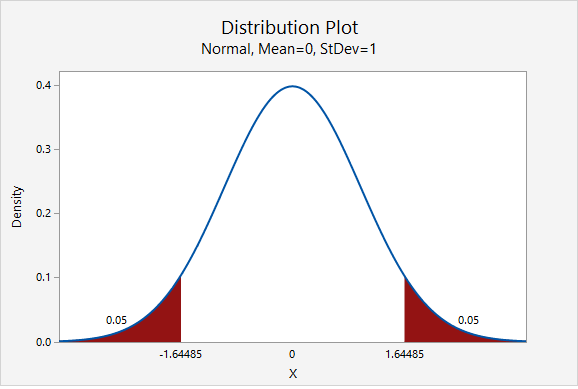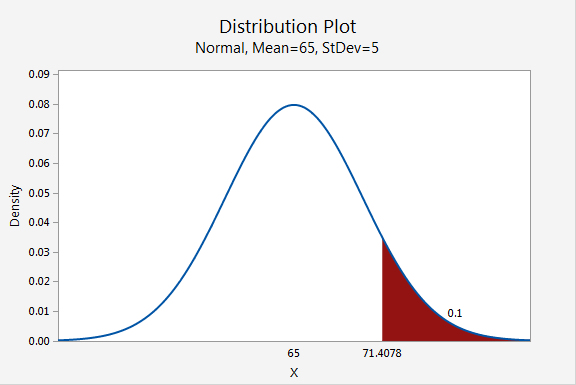# 7.3 - Minitab Express: Finding Values Given Proportions

Minitab Express can also be used to find the values that separate a given proportion of the normal distribution. This procedure can be used to find the z* multiplier when constructing a confidence interval for any confidence level. For example, the z* multiplier for a 90% confidence interval is the z value that separates the middle 90% from the outer 10%, which we see in the first example below.

In Minitab Express, we can also adjust the mean and standard deviation of the normal distribution to find the values on different types of normal distributions that separate given proportions, as seen in the second example below.

## MinitabExpress – Finding the Middle 90% of a z Distribution

Scenario: What z scores separate the middle 90% of the standard normal distribution from the outer 10%?

We will construct a standard normal distribution with a mean of 1 and standard deviation of 0. We will find the points that separate the middle 0.90 from the outer 0.10. Note that the outer 0.10 will be equally split between the two tails:

Steps
1. On a PC: from the menu select STATISTICS > Distribution Plot
On a Mac: from the menu select Statistics > Probability Distributions > Distribution Plot
2. Select Display Probability (Note: The default is the standard normal distribution)
3. Select A specified probability
4. Select Equal tails
5. For Probability enter 0.10

This should result in the following output:The z scores that separate the middle 90% of the distribution from the outer 10% are ±1.64485

Video Walkthrough

Select your operating system below to see a step-by-step guide for this example.

## MinitabExpress – Finding the Top 10% of Vehicle Speeds

Scenario: Vehicle speeds at a highway location have a normal distribution with a mean of 65 mph and a standard deviation of 5 mph. What speed separates the top 10% of vehicles?

We will construct a normal distribution with a mean of 65 and standard deviation of 5. We will find the point that separates the to 0.10 from the bottom 0.90:

Steps
1. On a PC: from the menu select STATISTICS > Distribution Plot
On a Mac: from the menu select Statistics > Probability Distributions > Distribution Plot
2. Select Display Probability
3. For Distribution select Normal
4. For Mean enter 65
5. For Standard deviation enter 5
6. Select A specified probability
7. Select Right tail
8. For Probability enter 0.10

Note: You could also select Left tail and enter 0.90 as the probability.

This should result in the following output:The speed that separates the top 10% is 71.4078 mph.

Video Walkthrough

Select your operating system below to see a step-by-step guide for this example.